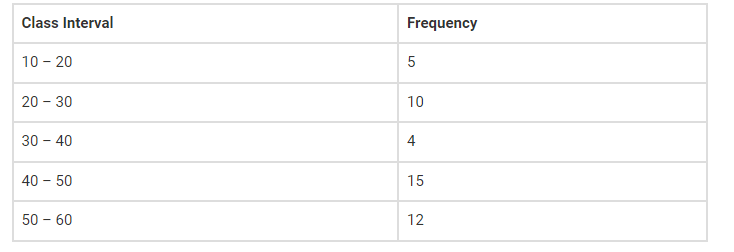# Given below is a frequency distribution table.

Question:

Given below is a frequency distribution table. Read it and answer the question that follow.(a) What is the lower limit of the second class interval?

(b) What is the upper limit of the last class interval?

(c) What is the frequency of the third class?

(d) Which interval has a frequency of 10?

(e) Which interval has the lowest frequency?

(f) What is the class size?

Solution:

(a) What is the lower limit of the second class interval?

The lower limit of the second class interval 20 – 30 is 20.

(b) What is the upper limit of the last class interval?

The upper limit of the last class interval 50 – 60 is 60.

(c) What is the frequency of the third class?

The frequency of the third class 30 – 40 is 4.

(d) Which interval has a frequency of 10?

Second class interval 20 – 30 has a frequency of 10.

(e) Which interval has the lowest frequency?

Third interval 30 – 40 has the lowest frequency i.e. 4.

(f) What is the class size?

The difference between the upper and lower limit of a class interval is called the Size of the class interval.

Size = Upper limit – Lower limit

= 20 – 10

= 10